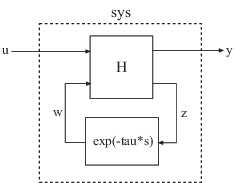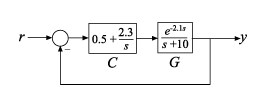# getDelayModel

State-space representation of internal delays

## Syntax

```[H,tau] = getDelayModel(sys) [A,B1,B2,C1,C2,D11,D12,D21,D22,E,tau] = getDelayModel(sys) ```

## Description

```[H,tau] = getDelayModel(sys)``` decomposes a state-space model `sys` with internal delays into a delay-free state-space model, `H`, and a vector of internal delays, `tau`. The relationship among `sys`, `H`, and `tau` is shown in the following diagram.```[A,B1,B2,C1,C2,D11,D12,D21,D22,E,tau] = getDelayModel(sys)``` returns the set of state-space matrices and internal delay vector, `tau`, that explicitly describe the state-space model `sys`. These state-space matrices are defined by the state-space equations:

• Continuous-time `sys`:

`$\begin{array}{c}E\frac{dx\left(t\right)}{dt}=Ax\left(t\right)+{B}_{1}u\left(t\right)+{B}_{2}w\left(t\right)\\ y\left(t\right)={C}_{1}x\left(t\right)+{D}_{11}u\left(t\right)+{D}_{12}w\left(t\right)\\ z\left(t\right)={C}_{2}x\left(t\right)+{D}_{21}u\left(t\right)+{D}_{22}w\left(t\right)\\ w\left(t\right)=z\left(t-\tau \right)\end{array}$`
• Discrete-time `sys`:

`$\begin{array}{c}Ex\left[k+1\right]=Ax\left[k\right]+{B}_{1}u\left[k\right]+{B}_{2}w\left[k\right]\\ y\left[k\right]={C}_{1}x\left[k\right]+{D}_{11}u\left[k\right]+{D}_{12}w\left[k\right]\\ z\left[k\right]={C}_{2}x\left[k\right]+{D}_{21}u\left[k\right]+{D}_{22}w\left[k\right]\\ w\left[k\right]=z\left[k-\tau \right]\end{array}$`

## Input Arguments

 `sys` Any state-space (`ss`) model.

## Output Arguments

 `H` Delay-free state-space model (`ss`). `H` results from decomposing `sys` into a delay-free component and a component `exp(-tau*s)` that represents all internal delays. If `sys` has no internal delays, `H` is equal to `sys`. `tau` Vector of internal delays of `sys`, expressed in the time units of `sys`. The vector `tau` results from decomposing `sys` into a delay-free state-space model `H` and a component `exp(-tau*s)` that represents all internal delays. If `sys` has no internal delays, `tau` is empty. `A,B1,B2,C1,C2,D11,D12,D21,D22,E` Set of state-space matrices that, with the internal delay vector `tau`, explicitly describe the state-space model `sys`. For explicit state-space models (E = I, or `sys.e = []`), the output `E = []`. If `sys` has no internal delays, the outputs `B2`, `C2`, `D12`, `D21`, and `D22` are all empty (`[]`).

## Examples

collapse all

Decompose the following closed-loop system with internal delay into a delay-free component and a component representing the internal delay.Create the closed-loop model `sys` from r to y.

```G = tf(1,[1 10],'InputDelay',2.1); C = pid(0.5,2.3); sys = feedback(C*G,1);```

`sys` is a state-space (`ss`) model with an internal delay that arises from closing the feedback loop on a plant with an input delay.

Decompose `sys` into a delay-free state-space model and the value of the internal delay.

`[H,tau] = getDelayModel(sys);`

Confirm that the internal delay matches the original input delay on the plant.

`tau`
```tau = 2.1000 ```